# Operations on Sets

Operations on Sets

In a class of 50 students, 35 said they loved reading, 30 said they loved painting.

The teacher wanted to know how many students loved both reading and painting, as well as those who did not have a hobby.

She grouped the students who had reading and painting into groups called sets.

Sets in math deals with a finite collection of objects, be it numbers, alphabets, or any real-world objects. Sometimes a necessity arises wherein we need to establish the relationship between two sets. There comes the concept of set operations.

In this lesson, we will learn the various notations of representing sets, how to operate on sets and their usage in real life.

Try out the set operations calculator to find the union, intersection, difference of sets! Enter the values in the set A and set B boxes and click on the 'Go' button to see the results.

## Lesson Plan

 1 What are Operations on Sets? 2 Important Notes on Operations on Sets 3 Tips and Tricks on Operations on Sets 4 Solved Examples on Operations on Sets 5 Interactive Questions on Operations on Sets

## What are Operations on Sets?

A set is defined as a collection of objects. Each object inside a set is called an 'Element'. Every element in a set is defined by the symbol 'e'. If it is not an element it is defined by e not.

A set can be represented in three forms. They are,

• Statement form
• Roster form
• Set builder form

### Statement Form

In the form of set representation, a statement describes the elements of a set.

For example,

The set of prime numbers from 1 to 20
Set of odd numbers from 1 to 100

### Roster Form

In this form of set representation, the elements of a set are listed inside curly brackets.

For example,

\begin{align} Set\: A \:= \{{1,2,3,4,5}\}\end{align}

\begin{align}Set\:B = \{{a,b,c,d,e}\}\end{align}

### Set Builder Form

In this form of set representation, a set is defined by a property about all the elements included in a set.

For example,

\begin{align} {\{ x\: : \:x\: is\: a\: even\: number\: less\: than\: 50 }\}\end{align}
\begin{align} {\{ x\: : \:x\: is\: a\: whole\: number\: less\: than\: 10 }\}\end{align}

Now let us learn the various operations on sets.

### Cardinality of a Set

The number of elements in a set defines the cardinality of a set. For a set A, cardinality is defined as n(A).

For example,

\begin{align} Set\: A \:= \{{1,2,3,4,5}\}\end{align}

Cardinality, \begin{align}n(A) = 5 \end{align}

### Venn Diagram

A venn diagram is a logical diagram that shows the possible relationship between different finite sets. It looks as shown below.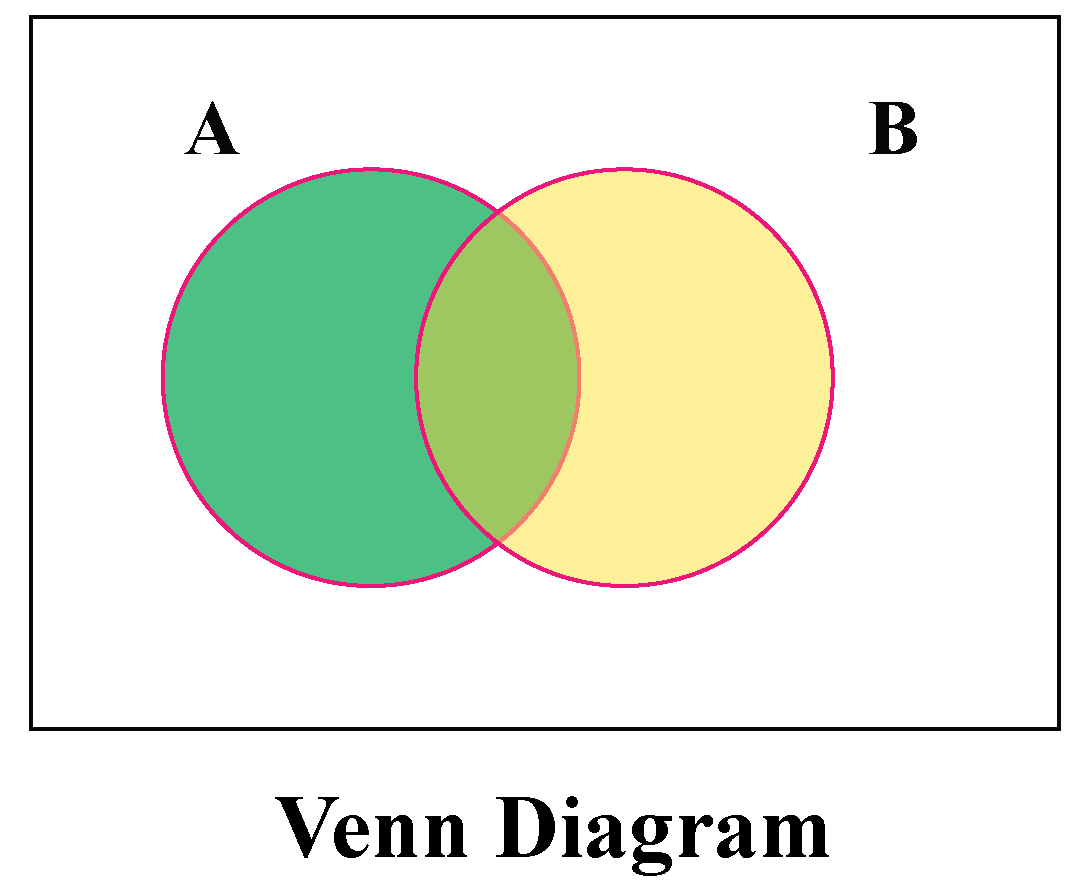## Basic Set Operations

### Union of Sets

For two given sets A and B, \begin{align} A\cup B\end{align} (Read as A union B) is the set of distinct elements that belong to set A and B or both.

Union of two sets can also be defined as

\begin{align}A\cup B = n(A) + n(B) - n(A\cap B)\end{align}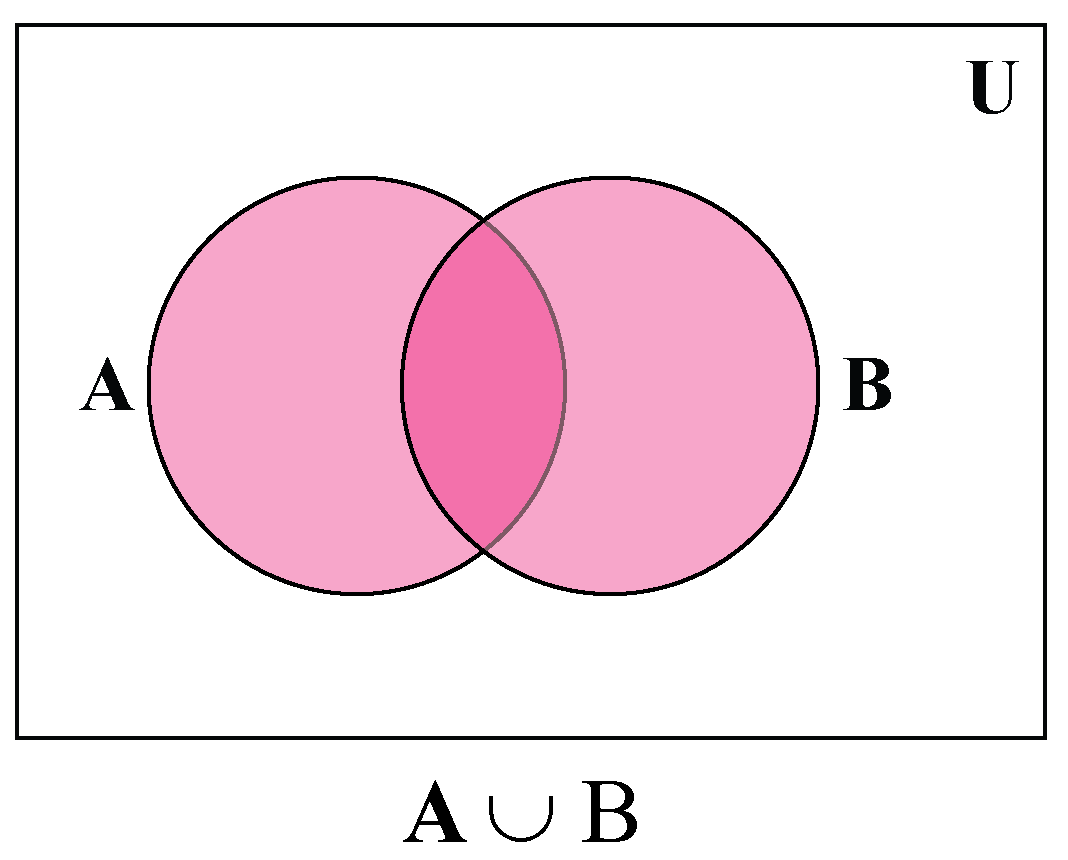For Example,

\begin{align} Set\: A = \{{1,2,3,4\}}\:\: Set\: B = \{{3,4,5,6\}}\end{align}

\begin{align} A\cup B = \{{1,2,3,4,5,6}\}\end{align}

### Intersection of Sets

For two given sets A and B, \begin{align} A\cap B\end{align} (Read as A intersection B) is the set of common elements that belong to set A and B.

Intersection of two sets can also be defined as

\begin{align}A\cap B = n(A) + n(B) - n(A\cup B)\end{align}

where \begin{align} n(A)\end{align} is the cardinality of set A,

\begin{align} n(B)\end{align} is the cardinality of set B,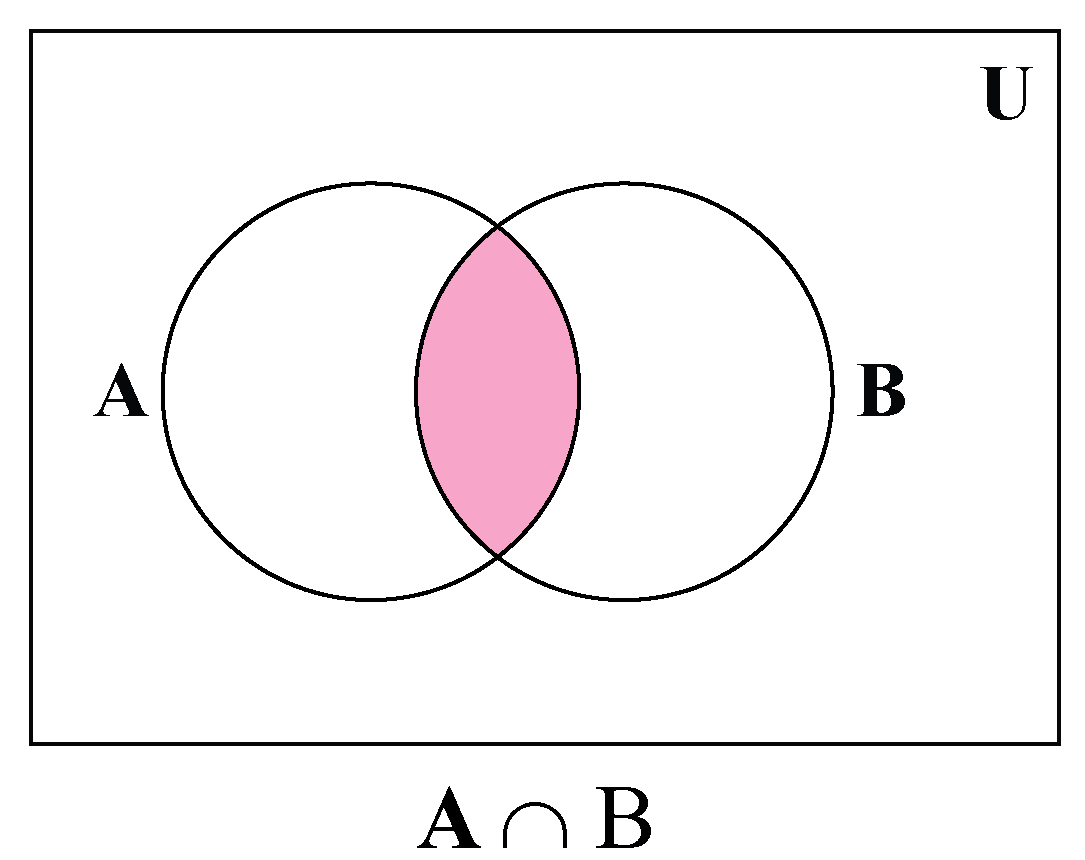For Example,

\begin{align} Set\: A = \{{1,2,3,4\}}\:\: Set\: B = \{{3,4,5,6\}}\end{align}

\begin{align} A\cap B = \{{3,4}\}\end{align}

### Disjoint Sets

Two sets A and B are said to be disjoint if there are no common elements, In other words, the intersection of the two sets is empty.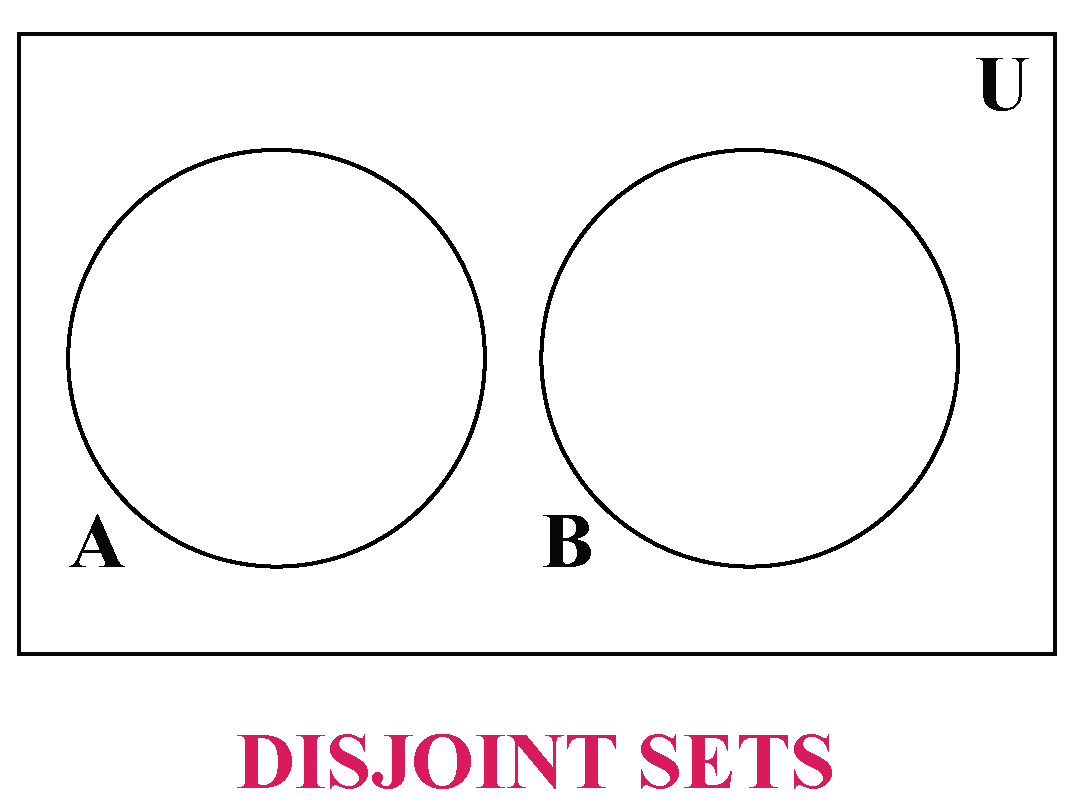For Example,

\begin{align} Set\: A = \{{1,2,3,4\}}\:\: Set\: B = \{{5,6,7,8\}}\end{align}

A and B are disjoint sets as they do not have any common elements.

### Set Difference

The difference between sets A and B \begin{align} A-B\end{align} lists all the elements in set A but not in set B.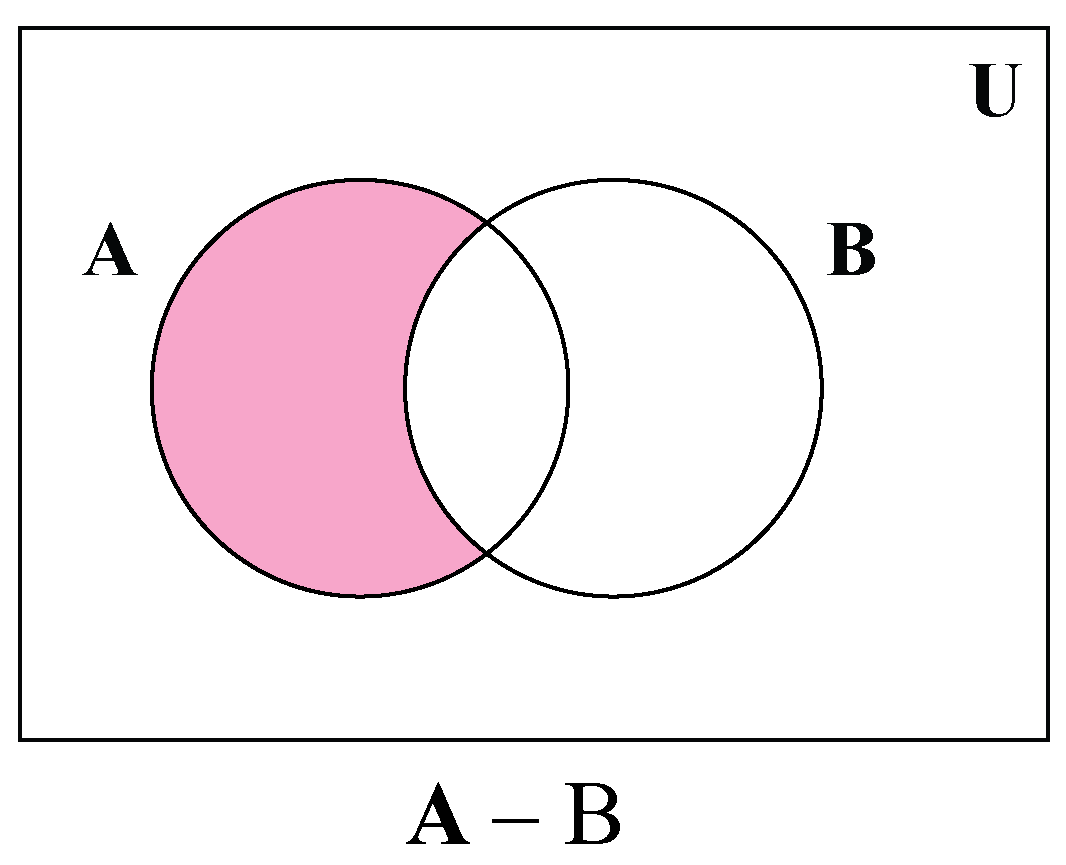For Example,

\begin{align} Set\: A = \{{1,2,3,4\}}\:\: Set\: B = \{{2,3,5,7\}}\end{align}

\begin{align} A-B\: = \:\end{align} \begin{align}\{{1,4}\}\end{align}

### Complement of Sets

Complement of a set A  \begin{align} A'\end{align}(Read as A complement) is defined as the set of all the elements in the given universal set(U) that are not present in set A.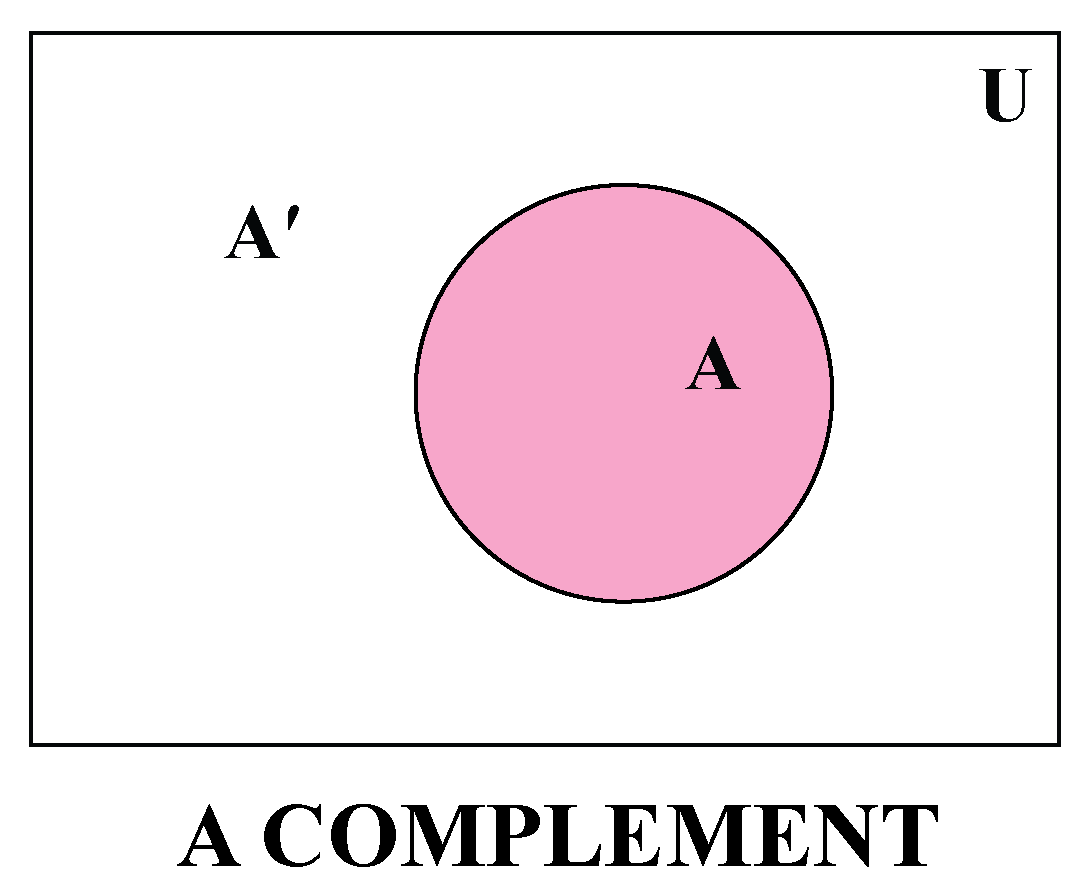For example,

\begin{align} Set \:U = \{{2,4,6,8,10,12}\}\end{align}

\begin{align}A = \{{4,6,8}\}\end{align}

\begin{align}A' = \{{2,10,12}\}\end{align}

## Properties of Operations of Sets

The important properies on operations of sets are stated below.

### Commutative Property of Sets

For any two given sets A and B, the commutative property is defined as,

\begin{align}A \cup B = B \cup A\end{align}

This means that union of two sets are commutative.

\begin{align}A \cap B = B \cap A\end{align}

This means that intersection of two sets are commutative.

### Associative Property of Sets

For any three given sets A,B and C the associative property is defined as,

\begin{align}(A \cup B) \cup C = A \cup (B \cup C) \end{align}

This means union of sets is associative.

\begin{align}(A \cap B) \cup C = A \cup (B \cap C) \end{align}

This means intersection of sets is associative.

### Distributive Property of Sets

For any three given sets, A,B and C the distributive property is defined as,

\begin{align}A \cap (B \cup C) = (A \cap B) \cup (A \cap C) \end{align}

Here intersection is distributed over union of sets B and C.

\begin{align}A \cup (B \cap C) = (A \cup B) \cap (A \cup C) \end{align}

Here the union is distributed over intersection of sets B and C.Tips and Tricks
1. The cardinality of a set denotes the number of elements in a set.
2. A Venn diagram can be used to establish the correct relationship between sets.
3. Each circle in a Venn diagram represents a set.

## Solved Examples

 Example 1

\begin{align}Set\: A = \{{a,b,c,d,e}\} \: Set \:B = \{{a,e,i,o,u}\} Set \: U = \{{a,b,c,d,e,f,g,h,i,j,k,l}\}\end{align}.

Find

a) \begin{align}A \cup B\end{align}
b) \begin{align}A \cap B\end{align}
c) \begin{align}A'\end{align}
d) \begin{align}A - B\end{align}

Solution

a) \begin{align}A \cup B = \{{a,b,c,d,e,i,o,u\}}\end{align}

b) \begin{align}A \cap B = \{{a,e\}}\end{align}

c) \begin{align}A' = \{{f,g,h,i,j,k,l}\} \end{align}

d) \begin{align}A - B = \{{b,c,d}\}\end{align}

 \begin{align}A \cup B = \{{a,b,c,d,e,i,o,u\}}\end{align}  \begin{align}A \cap B = \{{a,e\}}\end{align} \begin{align}A' = \{{f,g,h,i,j,k,l}\} \end{align}  \begin{align}A - B = \{{b,c,d}\}\end{align}
 Example 2

\begin{align}Set \:A =\{{2,4,6,8}\}, Set\:B = \{{4,5,6,7}\}, Set\:C = \{{6,8,10}\}\end{align}

Verify the result of

a) \begin{align}(A \cup B) \cup C = A \cup (B \cup C) \end{align}

b) \begin{align}A \cap (B \cup C) = (A \cap B)\cup (A \cap C) \end{align}

Solution

a) To verify this result, \begin{align}(A \cup B) \cup C = A \cup (B \cup C) \end{align}

Let us evaluate the left hand side, \begin{align}(A \cup B) \cup C \end{align}

\begin{align}A \cup B = \{{2,4,5,6,7,8}\}\end{align}

\begin{align}(A \cup B) \cup C= \{{2,4,5,6,7,8,10}\} ------- (1)\end{align}

Now let us evaluate the right hand side, \begin{align}(A \cup B) \cup C = A \cup (B \cup C) \end{align}

\begin{align}B \cup C = \{{4,5,6,7,8,10}\}\end{align}

\begin{align} A \cup (B \cup C) = \{{2,4,6,7,8,10}\} ------- (2)\end{align}

From equations (1) and (2) we conclude that,

\begin{align}(A \cup B) \cup C = A \cup (B \cup C) \end{align}

b) To verify this result, \begin{align}A \cap (B \cup C) = (A \cap B)\cup (A \cap C) \end{align}

Let us evaluate the left hand side, \begin{align}A \cap (B \cup C) \end{align}

\begin{align}B \cup C = \{{4,5,6,7,8,10}\}\end{align}

\begin{align}A \cap (B \cup C) = \{{4,6,8}\} ------- (1)\end{align}

\begin{align}A \cap B = \{{4,6}\}\end{align}

\begin{align}A \cap C = \{{6,8}\}\end{align}

\begin{align}(A \cap B)\cup (A \cap C) = \{{4,6,8}\} ---------(2) \end{align}

From equations (1) and (2) we conclude that,

\begin{align}A \cap (B \cup C) = (A \cap B)\cup (A \cap C) \end{align}

 Thus the results are verified.
 Example 3

In a school 200 students played football, 150 students played soccer and 100 students played both. Find how many students were there in the school?

Solution

Let us represent the number of students who played football as \begin{align}n(F) \end{align}

and

Number of students who played soccer as \begin{align}n(S) \end{align}

\begin{align}n(F) = 200\end{align}

\begin{align}n(S) = 150\end{align}

\begin{align}n(F \cap S) = 100\end{align}

We know that,

\begin{align}n(F \cup S) = n(F) + n(S) - n(F \cap S)\end{align}

Therefore,

\begin{align}n(F \cup S) = (200 + 150) - 100\end{align}

\begin{align}n(F \cup S) = 350 - 100\end{align}

\begin{align}n(F \cup S) = 250\end{align}

 The total number of students in the school were \begin{align} 250\end{align}
 Example 4

From a group of 400 people, 150 people liked swimming, 80 people liked both swimming and scuba-diving. How many scuba-diving?

Solution

Let us represent the number of people who liked swimming as \begin{align}n(S) \end{align}

and

Number of people who liked scuba-diving as \begin{align}n(D) \end{align}

and

the total number of people who liked both swimming and scuba-diving as  \begin{align}n(S \cap D)\end{align}

and

total number of people in the group as \begin{align}n(S \cup D)\end{align}

\begin{align}n(S) = 150\end{align}

\begin{align}n(D) = ? \end{align}

\begin{align}n(S \cup D) = 400\end{align}

\begin{align}n(S \cap D) = 80\end{align}

Number of people who liked scuba-diving is,

\begin{align}n(S \cap D) = n(S) + n(D) - n(S \cup D)\end{align}

\begin{align}80 = (150 + n(D)) - 400\end{align}

\begin{align}80 = n(D) - 250\end{align}

\begin{align}80 = n(D) - 250\end{align}

\begin{align}80 + 250 = n(D) \end{align}

\begin{align}330 = n(D) \end{align}

 The number of people who liked scuba-diving is \begin{align}330 \end{align}

Let's Summarize

The lesson targeted on the fascinating concept of operations of sets. By now you can find the solve questions on union, intersection, difference between two sets, and complement of sets.

At Cuemath, our team of math experts is dedicated to making learning fun for our favorite readers, the students!

Through an interactive and engaging learning-teaching-learning approach, the teachers explore all angles of a topic.

Be it worksheets, online classes, doubt sessions, or any other form of relation, it’s the logical thinking and smart learning approach that we, at Cuemath, believe in.

## FAQs on Operations on Sets

### 1. What are the 4 operations of sets?

Union, intersection, difference, complement are the 4 operations of sets.

### 2. What are the union and intersection of sets?

For any two sets A and B,  the union is defined as the combination of elements in both set A and B. Intersection of sets gives the common elements in set A and set B.

### 3. What is A U B in math?

In the set theory, 'U' is the symbol used to denote the union of two sets. For any two sets A and B, A U B defines the list of elements in set A and B.

### 4. How do we use sets in real life?

A set is a collection of elements. Some real-life examples of sets are a list of all the states in a country, a list of all shapes in geometry, list of all whole numbers from 1 to 100.

### 5. How do you solve set operations problems?

To solve set operation problems we use a Venn diagram to represent the relationship between the sets and apply the set operations formula for union, intersection, difference, or complement of a set.

### 6. What does \begin{align}\cap\end{align} mean?

In set theory '\begin{align}\cap\end{align}' is a symbol used to denote the intersection of two sets.

### 7. How do you find the difference between the two sets?

For any two sets A and B, the difference A - B lists all the elements in set A that are not in set B.

### 8. How do you find the complement of a set?

Given the universal set 'U' and set A, the complement of a set A is defined as the set of all elements in the universal set that are not present in set A.

More Important Topics
Numbers
Algebra
Geometry
Measurement
Money
Data
Trigonometry
Calculus